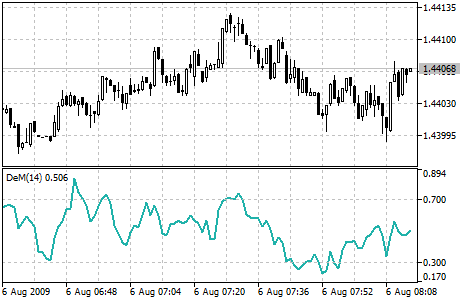Interesting script?
So post a link to it -
let others appraise it
You liked the script? Try it in the MetaTrader 5 terminal# DeMarker (DeM) - indicator for MetaTrader 5

Views:
15094
Rating:
Published:
2010.01.26 11:11
Updated:
2016.11.22 07:32

Demarker technical indicator (DeM) is based on the comparison of the period maximum with the previous period maximum.

If the current period (bar) maximum is higher, the respective difference between the two will be registered. If the current maximum is lower or equaling the maximum of the previous period, the naught value will be registered. The differences received for N periods are then summarized. The received value is used as the numerator of the DeMarker and will be divided by the same value plus the sum of differences between the price minima of the previous and the current periods (bars). If the current price minimum is greater than that of the previous bar, the naught value will be registered.

When the indicator falls below 30, the bullish price reversal should be expected. When the indicator rises above 70, the bearish price reversal should be expected.

If you use periods of longer duration, when calculating the indicator, you’ll be able to catch the long term market tendency. Indicators based on short periods let you enter the market at the point of the least risk and plan the time of transaction so that it falls in with the major trend.Demarker indicator

Calculation:

The value of the DeMarker for the "i" interval is calculated as follows:

DeMax (i) is calculated.

If HIGH (i) > HIGH (i - 1), then : DeMax (i) = HIGH (i) - HIGH (i - 1)
otherwise DeMax (i) = 0

DeMin (i) is calculated.

If LOW (i) < LOW (i - 1), then: DeMin (i) = LOW (i - 1) - LOW (i)

otherwise DeMin (i) = 0

The value of the DeMarker is calculated as:

DMark (i) = SMA (DeMax, N) / (SMA (DeMax, N) + SMA (DeMin, N))

where:

• HIGH (i) - the highest price of the current bar;
• LOW (i) - the lowest price of the current bar;
• HIGH (i - 1) - the highest price of the previous bar;
• LOW (i - 1) - the lowest price of the previous bar;
• SMA - Simple Moving Average;
• N - number of bars used for calculation.

Translated from Russian by MetaQuotes Software Corp.
Original code: https://www.mql5.com/ru/code/26Custom Moving Average

The Custom Moving Average is example of the Custom User Indicator - it calculates and shows the Moving Average.ColorLine

The ColorLine indicator shows the line of the price moving average on the chart. The line has diffrent colors (100 bars has the same color). The color settings of the line is changing every 5 ticks, there are 3 color schemes.Detrended Price Oscillator (DPO)

The Detrended Price Oscillator eliminates the trend effect of price movement. This simplifies the process of finding out cycles and overbought/oversold levels.Envelopes

The Envelopes Indicator is formed by two Moving Averages, one of them is shifted upward and another one is shifted downward. The selection of optimum relative number of band margins shifting is determined with the market volatility: the higher the latter is, the stronger the shift is.